# Team:Peking/Model

MODELING
Our model is integrated and tightly connected with experiments.

We applied ODE-system simulation to assist in recombinase characterization. The behavior of control units under recombinase-mediated sequence inversion was predicted with stochastic simulation. Furthermore, the generation of control unit design candidates was implemented with evolutionary algorithm. Screening of candidates was done with an original set of scoring rules. All these parts work closely together to realize the rational design of genetic sequential logic.

Also, the experimental viability of the design should be confirmed. We base our model on substantiated experiment observations. The sensitive parameters in ODE system for recombinase characterization were estimated with experiment data. In this process, the modeling and design are directed by experiments. Also, we predicted the operation range thus specifying RBS strength for expression of each recombinase. In turn, the modeling results aided in experiment design. Also, filtering criteria of control unit candidates have roots in experimental findings.

# Overview

Our model is integrated and tightly connected with experiments.

We applied ODE-system simulation to assist in recombinase characterization. The behavior of control units under recombinase-mediated sequence inversion was predicted with stochastic simulation. Furthermore, the generation of control unit design candidates was implemented with evolutionary algorithm. Screening of candidates was done with an original set of scoring rules. All these parts work closely together to realize the rational design of genetic sequential logic.

Also, the experimental viability of the design should be confirmed. We base our model on substantiated experiment observations. The sensitive parameters in ODE system for recombinase characterization were estimated with experiment data. In this process, the modeling and design are directed by experiments.

We predicted the operation range thus specifying RBS strength for expression of each recombinase. In turn, the modeling results aided in experiment design. Also, filtering criteria of control unit candidates have roots in experimental findings.

# Flip-flop

## Intention of the Model

According to our design of biological flip-flops, a recombinase-based device must be constructed, characterized and standardized. Recombinases can operate on sequences with recombination sites thus altering the direction of transcription. In this section we propose a model that characterize the process of gene recombination with ordinary differential equations (ODEs). Each reaction in the process is described with Hill or mas action kinetics. The aims of this model are listed as follows:
1. Estimate parameters in the model from experimental data and fine-tune the model.
2. Simulate the sequence recombination process via recombinases. Show the changing of quantities of original and flipped sequences over time. Prove that the device can work as expected.
3. Analyze the effects of varying recombinase production and degradation rates on the overall performance of the device.
4. Assist in designing of the genetic circuit.

## Biological Basis and Assumptions

The sequence-flipping device is based on the integrase family of recombinases. On this page we focus only on the Bxb1 serine integrase, but the same dynamics model and analysis applies to two other types TP901 and PhiC31. Mechanism of serine integrases is well recorded in literature.

### SET Process

After transcription and translation of the Bxb1 recombinase gene, the proteins are expressed. Two Bxb1 monomers form a dimer. In dimerized form, the recombinase proteins can bind to attL and attR binding sites around the constitutive promoter J23119 on the reporter sequence. When both sites are occupied, synapse between the two sites can happen. This can enable flipping of the sequence and alter the direction of the promoter. The flipping process changes the direction of the reporter promoter and transforms the attB and attP sites in attL and attR. In its initial direction, the promoter starts GFP transcription; after flipping, it turns to RFP. By measuring cell fluorescence with flow cytometry, we can quantify the ratio of flipped sequences (attL/R ratio). The data-fitting procedure relies on this key indicator.

### RESET Process with Integrase-RDF Fusion Protein

Another kind of recombinases - excisionases are able to recognize and bind attL and attR sequences. With the help of excisionases, the state transitions become reversible. Recombination Directionality Factors (RDF) are a kind of protein that achieves reverse flipping to recover the sequence flipped by recombinases. By co-expression of the integrase with the corresponding RDF, or expression of the integrase-RDF fusion protein, recombination between the attL and attR sites can be induced. Thus, the flip-flop can restore to the previous state.

## Model Composition

The following part describes species and reactions involved in different components of the model.

### Arabinose-Induced Protein Expression

We have decided to use an arabinose-induced promoter (pBAD) for the expression of recombinases on ColE1 vector. This promoter can be modelled as the following chemical system.

$$(AraB:AraC) \xrightarrow[]{K_{transcription}} mRNA \xrightarrow[]{K_{translation}} Recombinase$$
$$mRNA \xrightarrow[]{\gamma_{mRNA}} \emptyset$$
$$Recombinase \xrightarrow[]{\gamma_{prot}} \emptyset$$

The promoter pBAD binds to AraC and this represses transcription of mRNA. Arabinose will bind to AraC and form the Arab:AraC compound, allowing transcription to occur.

We assume that:
1. AraC is always in large concentration
2. AraC binding to arabinose occurs on a faster time scale to transcription
Therefore it is not necessary to consider individual concentrations of arabinose and AraC. We need only include the concentration of (Arab:AraC) complex in the model. The transcription rate constant K on the schematic diagram above is not a simple constant but described as a Michaelis-Mentin kinetics equation below.

$$\frac{\mathrm{d}[mRNA]}{\mathrm{d}t}=K_{max}\frac{[Arab:AraC]^n}{[Arab:AraC]^n+K_{half}^n}-\gamma_{mRNA}[mRNA]$$

Although pBAD system is known for rigidity, we observed leaky expression in the experiments. So we add a basal translation level to describe leakiness. The basal expression level should be several orders of magnitude lower than the maximal level.

$$\frac{\mathrm{d}[mRNA]}{\mathrm{d}t}=K_{basal}+K_{max}\frac{[Arab:AraC]^n}{[Arab:AraC]^n+K_{half}^n}-\gamma_{mRNA}[mRNA]$$

The mRNA degradation and dilution rates are combined into a single parameter. Protein (recombinase) synthesis and degradation can be modeled with the equation below.

$$\frac{\mathrm{d}[P]}{\mathrm{d}t}=\alpha_{Int}[mRNA]-\gamma_{Int}[P]$$

Again, we combine dilution and degradation rate of recombinase into a single parameter.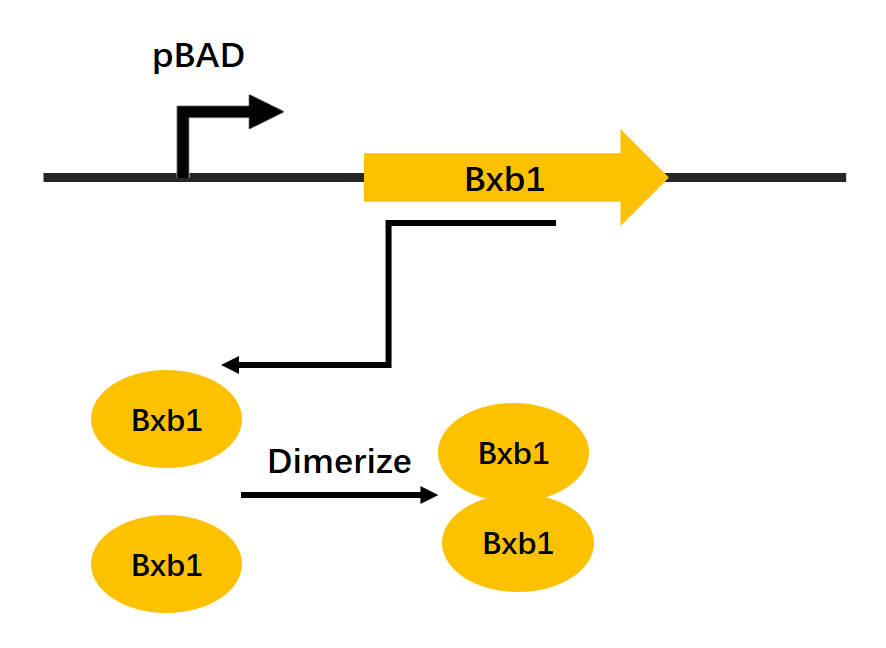Figure1. Recombinase expression mechanism schematic drawing

### Recombinase Functioning

Here we make the following assumptions about the reporter vector:
1. The total concentration of the reporter vector in cell remains constant during the whole reaction. This is based on the fact that reporter fluorescent proteins are on the stringent pSC101 vector, whose copy number is strictly controlled to around 5~10 nM. We assume this concentration to be 5nM.
2. The reporter sequences are divided into 4 states: B0F, B1F, B2F and R. Here F and R denote the direction of the constitutive promoter. F stands for “Forward”, corresponding to the initial direction (GFP direction) before flipping. R stands for “Reverse”, corresponding to the direction after the flipping (RFP direction). B0, B1 and B2 denote the number of recombination sites bound by the recombinase dimer.
3. After 12h of reaction, the RFP expressed by the promoter in reverse direction have all matured. The efficiency of the recombinase flipping mechanism is defined as the cell population fraction expressing RFP in FACS data. See Experiment Part for detailed calculation

The following diagram summarizes the mechanism and key reactions in the recombinase flipping mechanism.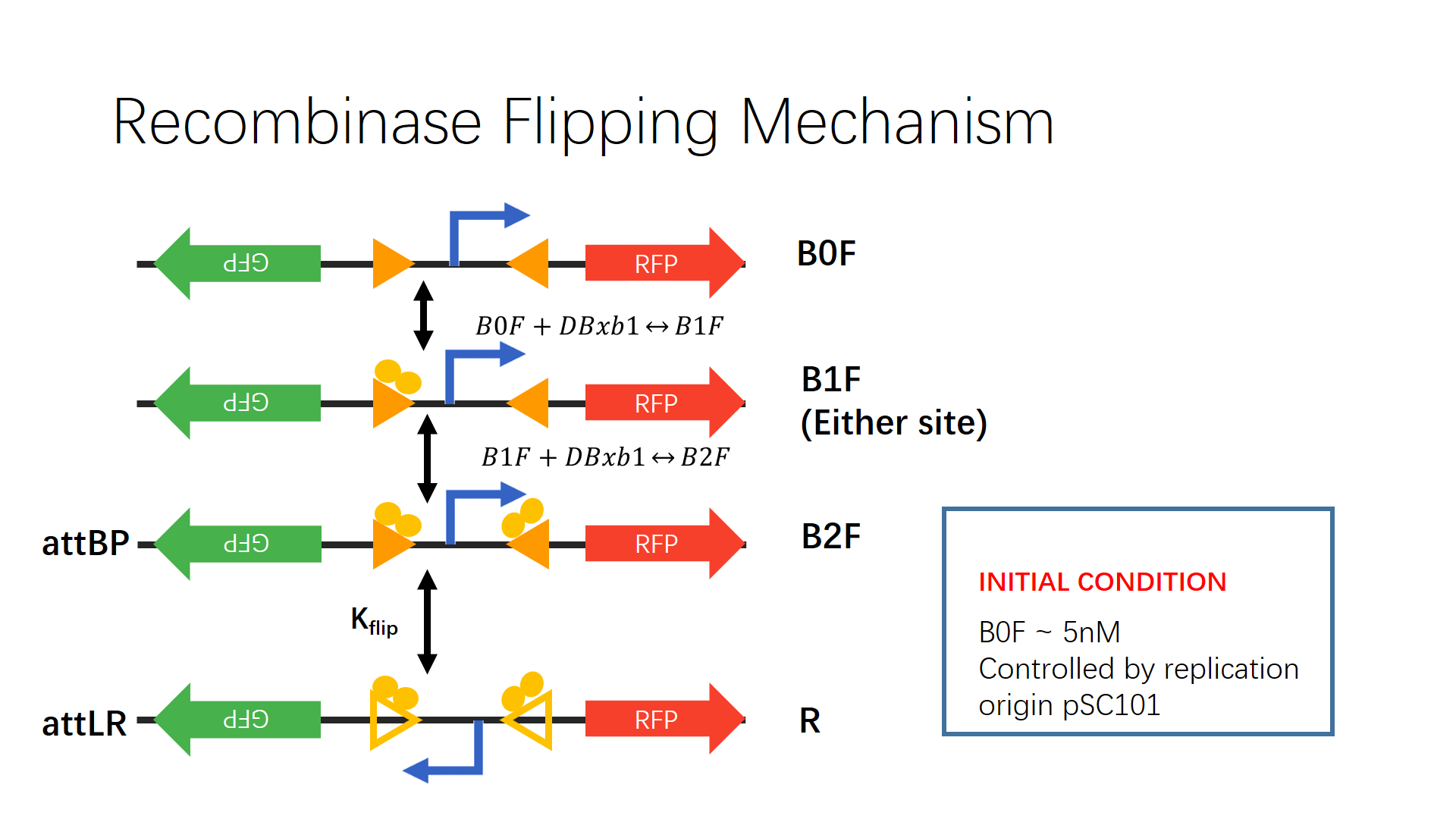Figure2. Recombinase flipping mechanism schematic drawing

The table below are reactions and parameters involved in this model.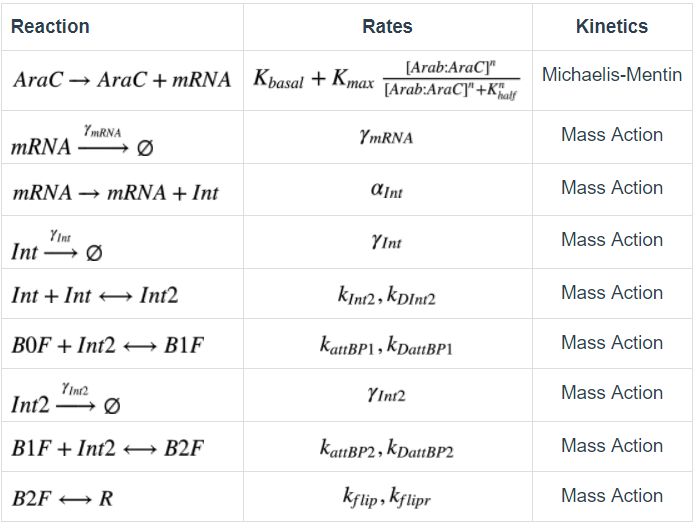Table1. Recombinase flipping mechanism reaction and parameter table Simbiology

These reactions, together with kinetic laws and reaction rate parameters, can be transformed into an ordinary differential equation (ODE) system.

### Recombinase-RDF Fusion Protein Functioning

Similar mechanisms can describe recombinase-RDF fusion protein functioning. The recombinases themselves cannot flip back the sequence flanked between attL and attR sites. They have to work together with recombination directionality factors (RDFs) to accomplish this task. Several proposals on genetically engineered RDF expression were suggested by Bonnet and colleagues.  In our experiments, we implemented the fusion protein construction, which was also reported to be functional in other work. 
We make the following assumptions about recombinase-RDF fusion proteins:
1. The recombinase-RDF fusion proteins have the similar transcription and translation behavior as the recombinases
2. The binding and unbinding of recombinase-RDF fusion proteins to attL and attR sites are reversible reactions
3. Flipping of the target sequence is also reversible

## Simulation Results

### Simulation Tool and Methods

We used MATLAB Simbiology to convert the chemical reaction system into an ODE system. This can be done through building the reaction pathway in a graphical interface and specify the parameters. Tasks as simulation, sensitivity analysis and parameter scanning can then be deployed. Below is the diagram that represents our ODE system model. Yellow circles represent reactions and blue ovals represent species.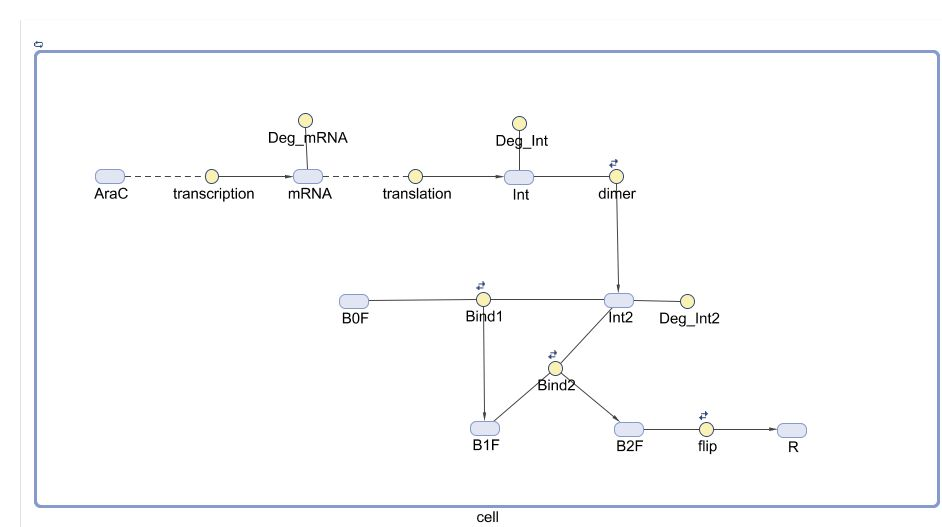Fig.3. Graphical representation of ODE system in MATLAB Simbiology

Reaction rate parameters for pBAD transcription, mRNA degradation, integrase dimerization and degradation are recorded in literature. Our goal is to use experimental data to estimate the following parameters:
1. Association constant between Integrase dimer and attB/P recombination sites: $$k_{attBP}, k_{DattBP}$$ (We assume that two binding parameters are the same, $$k_{attBP1}=k_{DattBP2}$$)
2. Flip reaction rate (forward and reverse) $$k_{flip}, k_{flipr}$$
3. Integrase translation rate $$\alpha_{Int}$$. This parameter is directly correlated with the RBS of the integrase, which is tuned through experiment（See here for details).

Thus, we arrive at a 14-parameter ODE system, with 5 parameters sensitive. We started from values described in literature and used constraint optimization using derivative-based method (fmincon) to do further estimation. Simulation was done through the ODE23t solver (Mod. Stiff/Trapezoidal) in MATLAB.

### Recombinase Dynamics

Here we show the time-course variation of the species concentration under two conditions: 0 and 10nM Arabinose induction. Experimental data of Bxb1_F8 listed below.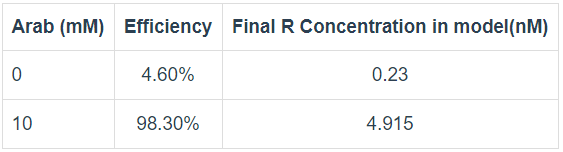Table2. Experiment observation of recombinase flipping reaction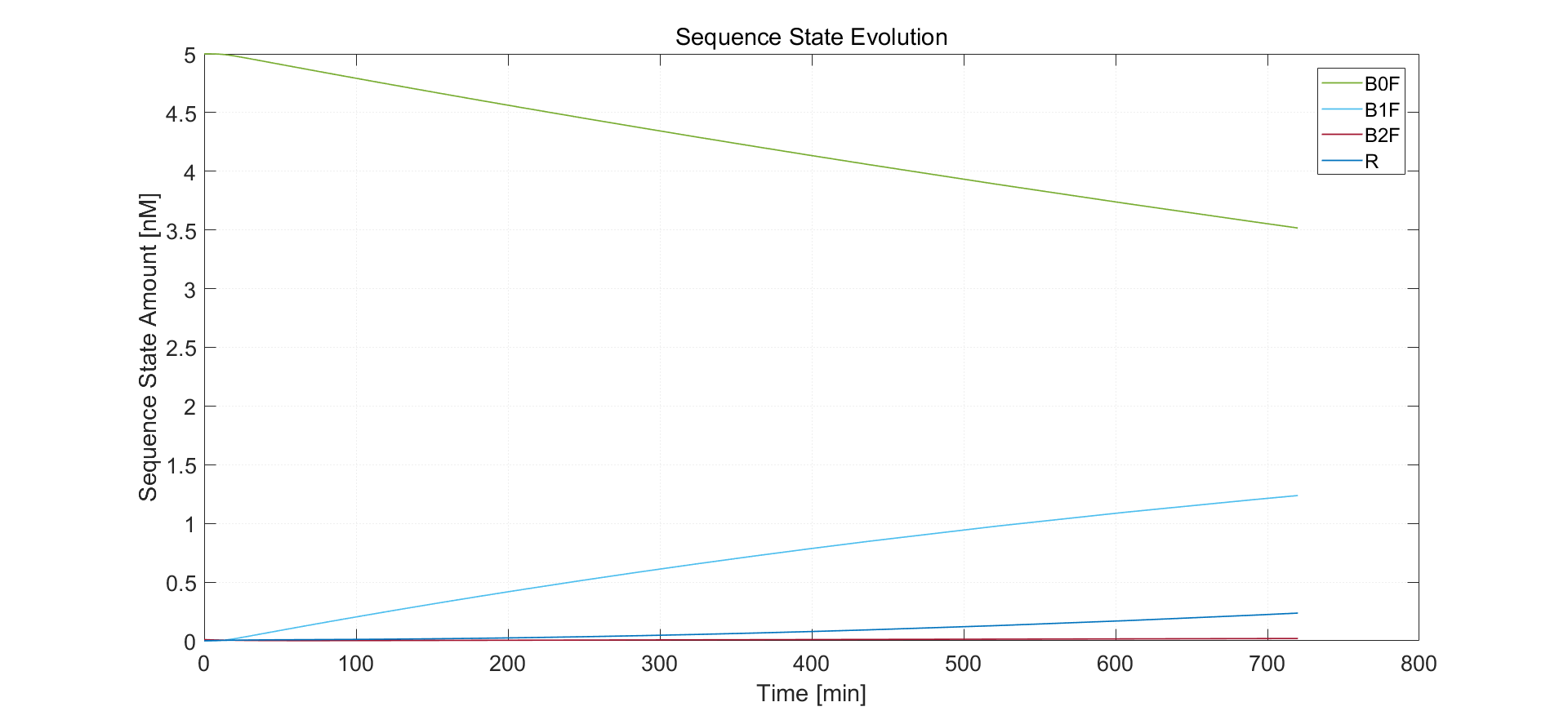Fig.4. Simulation result of no arabinose induction

We expect rather low expression of recombinase protein, which is shown in the simulation result. Almost no sequence has both sites bound with recombinase dimers. Sequence of type R has final concentration 0.23 nM, which is identical to the experimental results.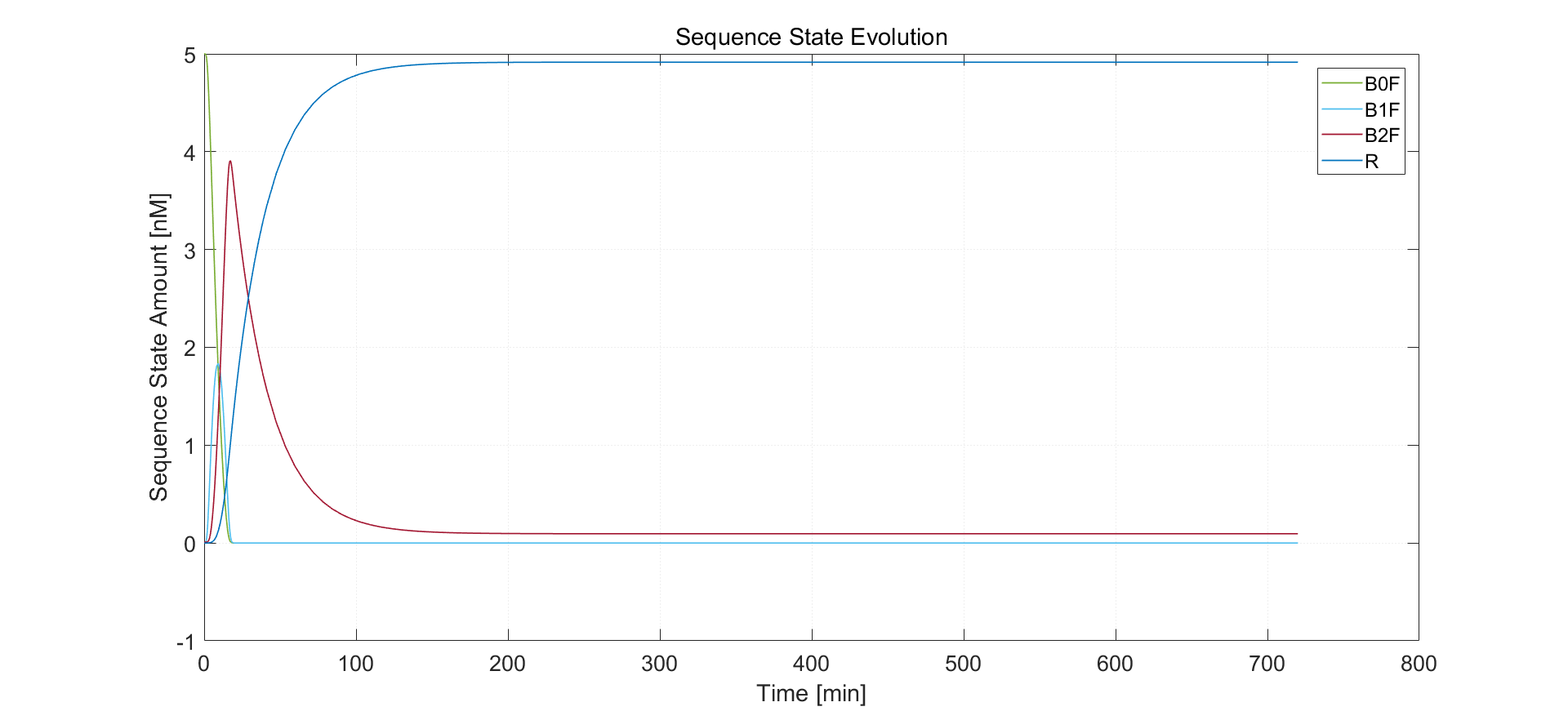Fig.5. Simulation result of 10nM arabinose induction

Under 10nM arabinose induction, sequence of type R has final concentration 4.916 nM, which also fits with experimental results. Sequence states stabilize after approximately 3 hours. After nearly 2 hours, sequence in B2F state has all been flipped.

### Sensitivity Analysis

In order to determine which parameters of the system can be tuned for our purpose, we perform a sensitivity analysis towards the possible sensitive parameters. This allows us to indentify the parameters that have the greatest influence on the final state of the system.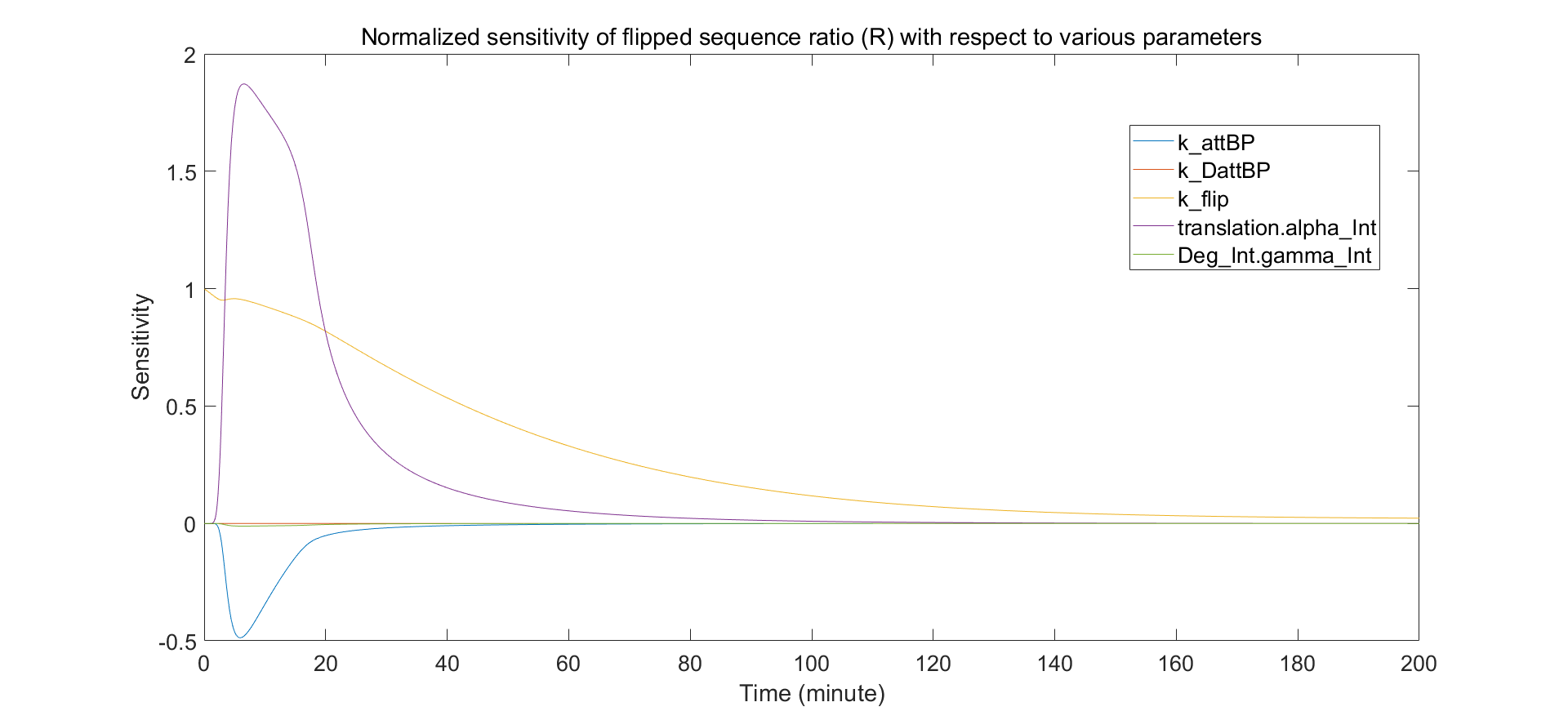Fig.6. Sensitivity of the promoter switching with respect to the main parameters

The integrase RBS strength ($$\alpha_{Int}$$) and the strength of the integrase flipping rate ($$k_{flip}$$) are biologically tunable parameters. Flipping rate can be altered by selection of integrase. These parameters have significant influence on the response of the switch to activation.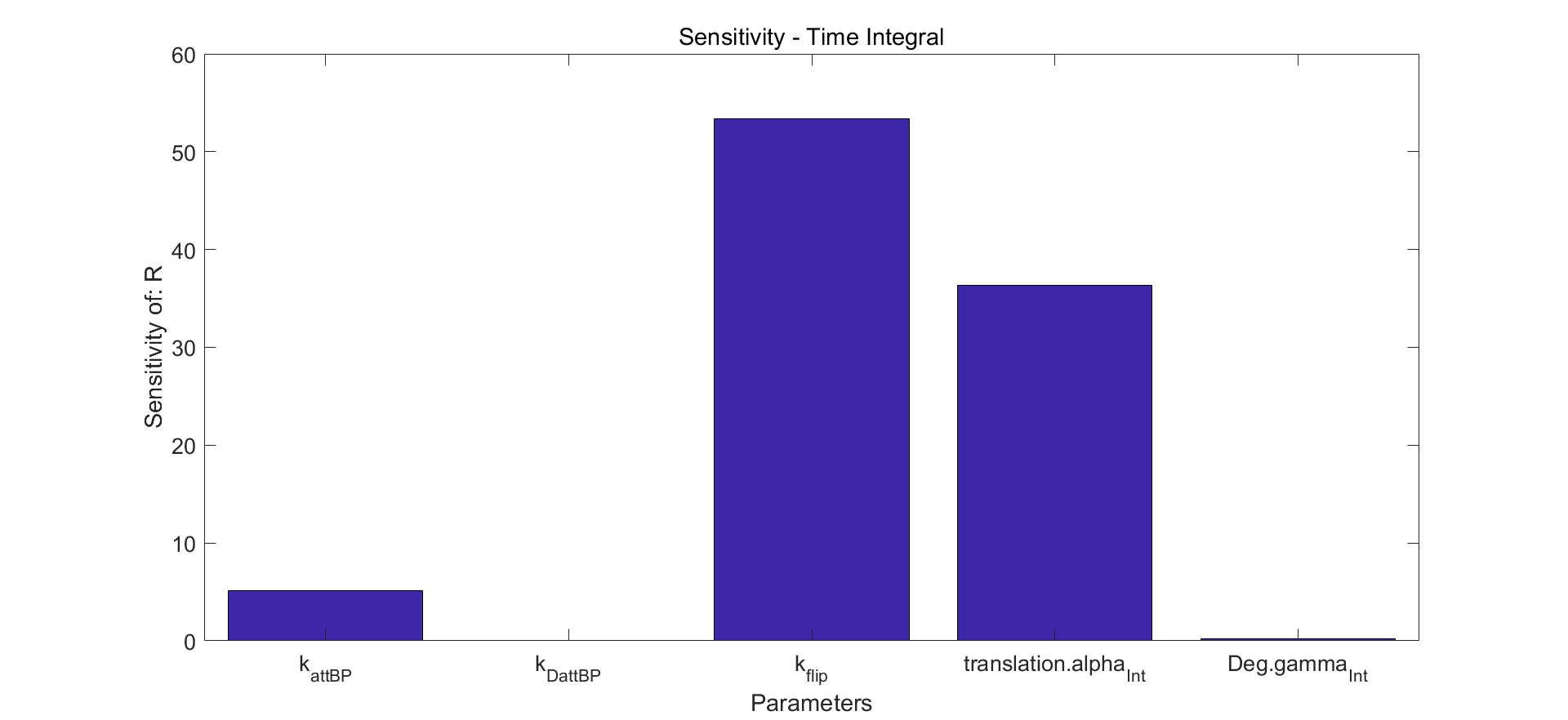Fig.7. Time integral results of sensitivity analysis.

### Translation and Degradation Operative Range

The aim of the experiment in recombinase characteristics and standardization is to find the best RBS-recombinase pair. In the model, this means determining the operative range of two parameters: the recombinase flipping rate and translation rate.
We made the heat map of the final R state concentration under varying $\alpha_{Int}$ and $k_{flip}$. Results show that recombinase devices have a rather wide functional range. Failure to generate enough flipped sequences can happen only when one of the two parameters is low.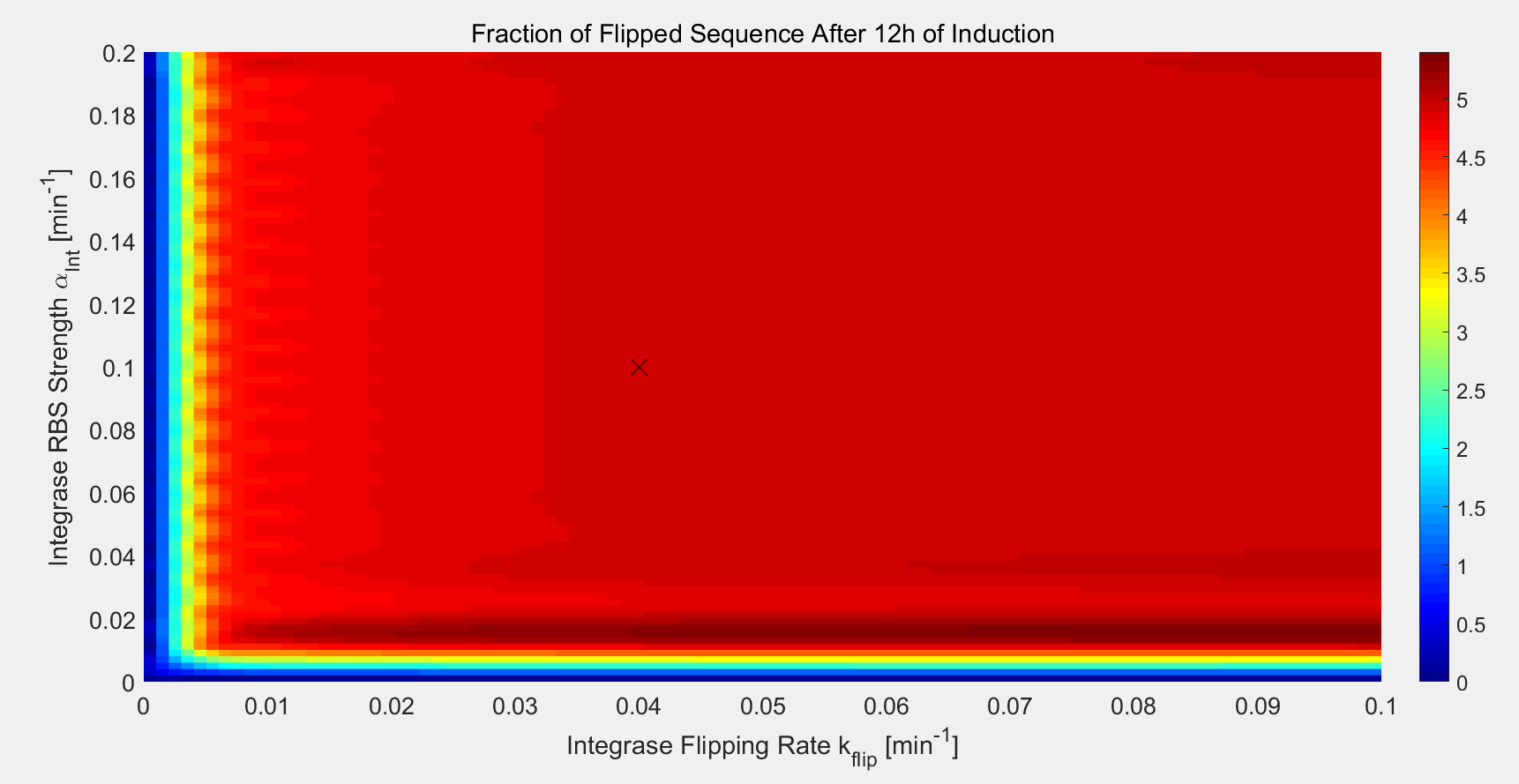Fig.8. Functional regions of the parameter space with efficiency

A cross is put on the point of Bxb1-F8 experiment in the above simulations

### Assembly

We assembled the two recombinases and recombinase-RDF fusion proteins as the structure of our design. The bio-flip-flop is constructed as two sequences each with an invertible promoter flanked between recombination sites and expressing recombinase or recombinase-RDF fusion protein mediating inversion of another sequence.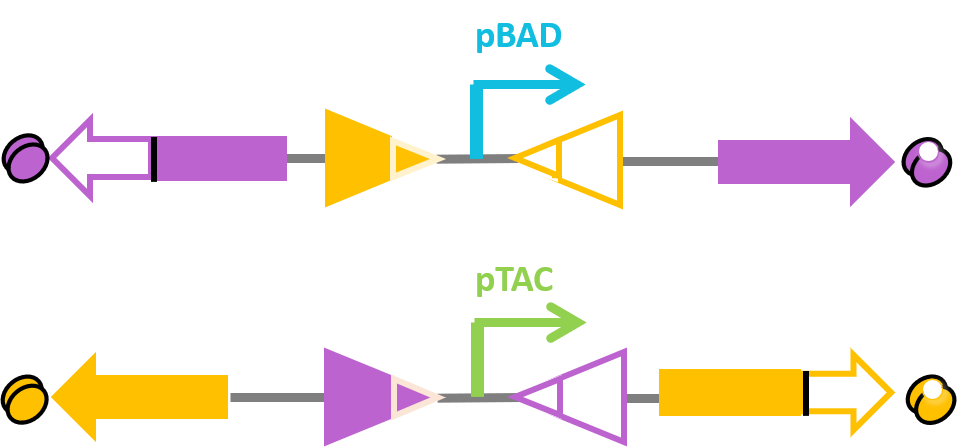Fig.9. Schematic drawing of bio-flip-flop structure. Recombinases, recombinase-RDF fusion proteins and their corresponding sites are in the same color. X_F denotes the fraction of forward direction (right) of the upper promoter pBAD. Y_F denotes the fraction of forward direction (right) of the lower promoter pTAC.

Then we did simulation of a whole process of bio-flip-flop with four flipping reactions happening in sequential order induced by four temporally adjacent inducing events. Parameters were estimated from experimental data. Inducing of the system is in the form of square waves to simulate manual induction like changing of medium.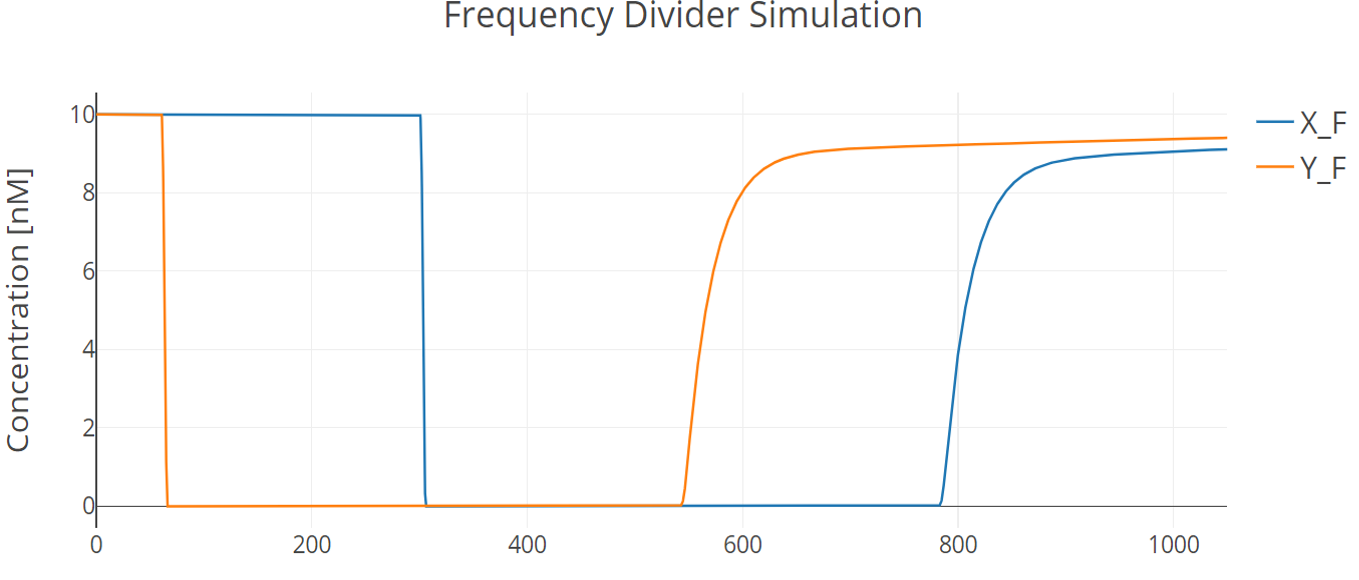Fig.10. Simulation result of the whole bio-flip-flop process

Induction on X promoter (pBAD) happens at 60min and 540min, lasting 180min each. Induction on Y promoter (pTAC) happens at 300min and 780min, lasting 180min each. Every state is held for 1h.

## References

1. Singh, S., Ghosh, P., & Hatfull, G. F. (2013). Attachment site selection and identity in Bxb1 serine integrase-mediated site-specific recombination. PLoS genetics, 9(5), e1003490.
2. Manen, D., & Caro, L. (1991). The replication of plasmid pSC101. Molecular microbiology, 5(2), 233-237.
3. Sourjik, V.,& Berg, H. C. (2004). Functional interactions between receptors in bacterial chemotaxis. Nature, 428(6981), 437.
4. Rosano, G. L., & Ceccarelli, E. A. (2014). Recombinant protein expression in Escherichia coli: advances and challenges. Frontiers in microbiology, 5.
5. Lewis, J. A., & Hatfull, G. F. (2001). Control of directionality in integrase-mediated recombination: examination of recombination directionality factors (RDFs) including Xis and Cox proteins. Nucleic acids research, 29(11), 2205-2216.
6. Bonnet, J., Subsoontorn, P., & Endy, D. (2012). Rewritable digital data storage in live cells via engineered control of recombination directionality. Proceedings of the National Academy of Sciences, 109(23), 8884-8889.
7. Olorunniji, F. J., McPherson, A. L., Rosser, S. J., Smith, M. C., Colloms, S. D., & Stark, W. M. (2017). Control of serine integrase recombination directionality by fusion with the directionality factor. Nucleic acids research, 45(14), 8635-8645.

# Controller

## Intention of the Model

In this model, we aim to describe the sequence modification process of the control unit sequence with recombination sites. Different recombinases are expressed in series to alter the direction of terminators between corresponding recombination sites on the control unit circuit. The combination of unidirectional terminators in different directions (reverse or foward) determines the state of RNA polymerase flux, thus controlling expression of the downstream gene.

Though we have done simulation of recombinase dynamics in the previous section, it is necessary to consider two key concepts of biological processes: 1. population behavior 2. stochastic process

The reason for modeling cell population behavior is that deployment of synthetic bacterial devices would certainly involve populations of cells. Furthermore, we demonstrate our design through continuous imaging of entire cell cultures. This is a theory-experiment interaction procedure. The reason for stochastic modeling is self-evident. Cellular biological processes, especially gene expression, are innately noisy and stochastic. Previous work has demonstrated the effectiveness of the integration of population-level dynamics and stochastic cellular responses to inputs.It is important to explain how stochastic single-cell responses affect overall population-level distributions and behavior.

This section describes a Markov model of recombinase-based DNA flipping and a Gillespie stochastic simulation method to observe theoretical sequence state evolution. We will focus on our demonstration design, which can be generalized to other designs.

## Biological Basis

The control units are implemented experimentally by transforming a plasmid into an E. coli. This plasmid has similar structure as the reporter plasmid in the previous section. However, in this situation the sequence fragments being inverted are terminators. Using experimentally screened uni-directional terminators, the transcription or polymerase flux4 can be precisely controlled. The figure below illustrates the result of the design software.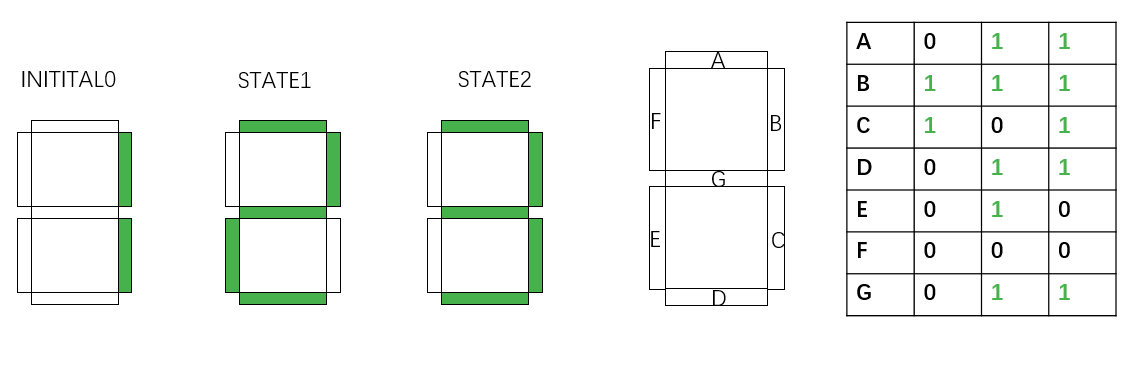In the seven-segment decoder tube demonstration, if we would like to display “1-2-3” counting, 3 kinds of control units have to be transformed into 3 strains of bacteria. 2 of them has only one pair of recombination sites, and only undergoes recombinase inversion once. The other one has two nested recombination sites. Successive inversion results in the same state as the initial one.

## Model Composition and Assumptions

A Markov process is defined upon a specific state space. We define that each state occupies a specific state. Then we can use Markov models to track cell state changes over time. This “state” consists of two parts: the state of recombination sites on the control unit sequence and the concentration or molecule number of two types of recombinases A and B. This definition was first proposed by Victoria Hsiao and colleagues to model a population-based event detector.5 The state of a single cell is defined by the DNA state and the copy numbers of integrases A and B. We denote the state of a single cell by (DNA, IntA, IntB).

### Cell States

All of the four possible DNA states are: the initial state ($$S_{init}$$), the IntA inversion only state ($$S_a$$), the IntB inversion only state ($$S_b$$), and the IntA then IntB inversion state ($$S_{ab}$$). We omit the state IntB then IntA inversion state ($$S_{ba}$$) because in our design and experiments, integrase expression is always induced in order. IntB expression can never be triggered before that of IntA. These states and transitions by recombinase inversions are illustrated below.

Our implementation requires that the control unit sequence on a stringent, low copy number plasmid. Thus we assume that each cell only has one copy of the control unit. Uni-directional terminators are screened by the criterion that the forward terminator strength exceeds the reverse one by a few orders of magnitude. This allows us to assume that when the terminator is in the forward direction, it can completely block RNA polymerase, while in the reverse direction, it cannot function(Click for more details).

### Integrase Production and Tetramerization

Serine integrases are produced as monomers that form dimers, search for specific attB and attP sequences, and, once both attB and attP sites are occupied, form a tetramer (dimer of dimers) that digests, flips and re-ligates the DNA. In this model we also consider leaky expression of the recombinases, because of observation of such phenomena in recombinase characterization experiments. Furthermore, induction of recombinase expression happens in order and we assume that at each time point, only one of the two inducers (a and b) exists. Thus we define the following table of state transitions and parameters: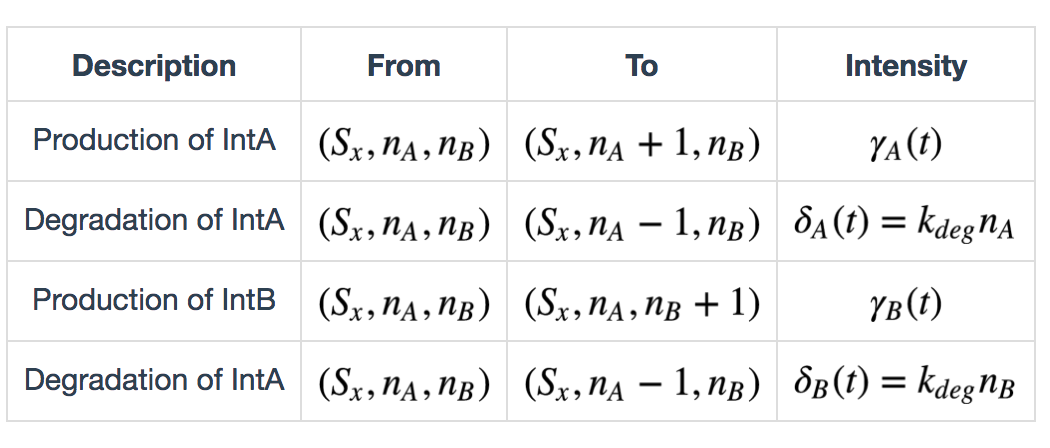$$x \in \{init, a, b, ab\})$$, $$\gamma_A(t)$$ and $$\gamma_B(t)$$ are defined as follows: $\gamma_A(t) = \begin{cases} k_{prodA} + k_{leakA}, & \text{if inducer a exists} \\ k_{leakB}, & \text{otherwise} \end{cases}$ $\gamma_B(t) = \begin{cases} k_{prodB} + k_{leakB}, & \text{if inducer a exists} \\ k_{leakB}, & \text{otherwise} \end{cases}$

The serine integrases need to tetramerize prior to DNA recombination. Each DNA binding site (attB and attP) is bound by two copies of integrase monomers in an independent manner to form a dimer. Once both of the binding sites on both DNA attachment sites are occupied, a dimer of the dimers (tetramer) is formed and recombination can occur. Here we deduce the reaction propensity of the tetramerization process. We only focus on the number of integrase molecules inside the cell, and ignore the detailed process of transcription and translation, and represent the process with a single parameter.

$$S_x:Int_i (i = 1,2,3,4) (x \in \{init, a, b, ab\})$$ denote the DNA-integrase complexes where $$i$$ denote the number of integrase molecules bound to DNA sequence. DNA recombination occurs when the complex state is at $$S_x:Int_4$$, which means the tetramerization of integrases on the DNA. Because of independent binding of DNA sequence with integrase dimers, the transition of states can be modeled by the following equations: $S_x \rightleftarrows S_x:Int_1 \rightleftarrows S_x:Int_2 \rightleftarrows S_x:Int_3 \rightleftarrows S_x:Int_4$
where the binding rate of DNA recombination sites (attB, attP) and integrase dimers is $$k_{bind}$$ (reaction from left to right side) and unbinding rate is $$k_{unbind}$$. The dynamics can be modeled by the following ordinary differential equations. $$\mathbb{P}_t(S_x:Int_i, n_*) (* = A, B)$$ is the joint probability distribution.

$\frac{d}{dt}\mathbb{P}_t(S_x, n_*) = -k_{bind}n_*\mathbb{P}_t(S_x, n_*) + k_{unbind}\mathbb{P}_t(S_x:Int_1, n_*)$ $\frac{d}{dt}\mathbb{P}_t(S_x:Int_1, n_*-1) = -(k_{bind}(n_*-1)+k_{unbind})\mathbb{P}_t(S_x:Int_1, n_*-1) + k_{bind}n_*\mathbb{P}_t(S_x, n_*) + k_{unbind}\mathbb{P}_t(S_x:Int_2,n_*-2)$ $\frac{d}{dt}\mathbb{P}_t(S_x:Int_2, n_*-2) = -(k_{bind}(n_*-2)+k_{unbind})\mathbb{P}_t(S_x:Int_2, n_*-2) + k_{bind}(n_*-1)\mathbb{P}_t(S_x:Int_1, n_*-1) + k_{unbind}\mathbb{P}_t(S_x:Int_3,n_*-3)$ $\frac{d}{dt}\mathbb{P}_t(S_x:Int_1, n_*-3) = -(k_{bind}(n_*-3)+k_{unbind})\mathbb{P}_t(S_x:Int_3, n_*-3) + k_{bind}(n_*-2)\mathbb{P}_t(S_x:Int_2, n_*-2) + k_{unbind}\mathbb{P}_t(S_x:Int_4,n_*-4)$ $\frac{d}{dt}\mathbb{P}_t(S_x:Int_4, n_*-4) = -k_{unbind}n_*\mathbb{P}_t(S_x:Int_4, n_*-4) + k_{bind}(n_*-3)\mathbb{P}_t(S_x:Int_3, n_*-3)$

We assume that binding and unbinding of integrase molecules to DNA equilibrate fast enough compared to the dynamics of DNA recombination, and the production and degradation of integrases (See Parameter Supplemental) and hence the above equations converge to an equilibrium while $$n_*$$ remain constant (equilibrium approximation). Let the above equations all equal to zero and solve in terms of $$\frac{d}{dt}\mathbb{P}_t(S_x:Int_4, n_*-4)$$, we can write:

$\frac{d}{dt}\mathbb{P}_t(S_x:Int_4, n_*-4) = \frac{n_* (n_*-1)(n_*-2) (n_*-3)}{K_{d*}^4 + K_{d*}^3 n_* + K_{d*}^2 n_* (n_*-1) + K_{d*}n_*(n_*-1)(n_*-2) + n_* (n_*-1)(n_*-2)(n_*-3)}$ ($$K_{d*} = k_{unbind}/k_{bind}$$ )

### Continuous-time Markov Process

Rather than accounting fro all individual DNA-integrase interactions, we have created a minimal model of stochastic transitions. Here only the final DNA states $$(S_o, S_a, S_b, S_{ab})$$ and the number of integrase monomer molecules (IntA, IntB) are tracked and all integrase activity is encompassed in the $$k_{flip*} (*=A,B)$$ term. Because no cooperativity has been observed in Bxb1 or TP901-1 DNA binding, e.g. occupation of attB does not increase the probability of attP binding, we assume that the flipping activity is zero unless at least four molecules are present. Then, the propensity function for state transitions is a function of integrase concentration (equivalent to protein molecule number in cell, if cell volume is assumed to be constant):

$\alpha_i(Int_*) = k_{flip*}(\frac{n_* (n_*-1)(n_*-2) (n_*-3)}{K_{d*}^4 + K_{d*}^3 n_* + K_{d*}^2 n_* (n_*-1) + K_{d*}n_*(n_*-1)(n_*-2) + n_* (n_*-1)(n_*-2)(n_*-3)})$ $$i = 1, 2, 3; * = A, B$$

Then the table of state transition and intensities can be completed. We consider three kinds of state transitions as described previously: $$S_{init}$$ to $$S_a$$, $$S_{init}$$ to $$S_b$$, and $$S_a$$ to $$S_{ab}$$. $$S_{init}$$ to $$S_b$$ is due to missing of integrase A flipping. This transition denotes the system’s ineffective fraction.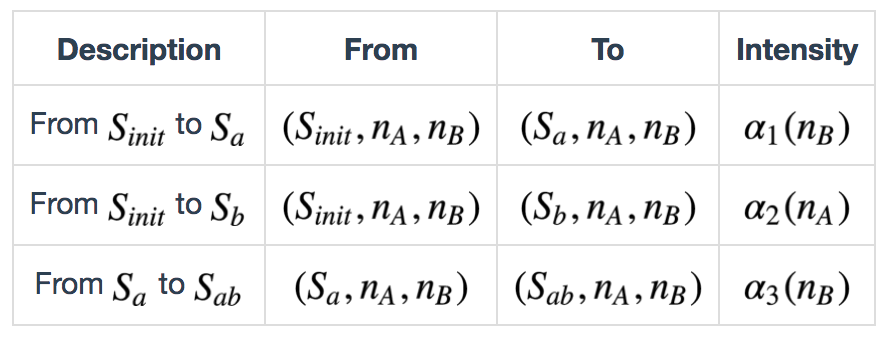Numbers of cells occupying each state are calculated by the summing equation below: $S_{i} = \sum_{n_A=0}^{\infty}\sum_{n_B=0}^{\infty} S(i,n_A,n_B), i \in \{S_{init},S_a,S_b\}$ because we only concentrate on the cell numbers, not the specific protein copy numbers in cells.

## Simulation Results

### Simulation Setting

In the simulation in this section, we consider induction with step inputs, where the inducers are either present or not present. Concentrations of the inducers when they are ‘’on’’ will be held constant. Also, it is important to note that inducer $$a$$ is still present during and after time $$\Delta t$$ when inducer $$b$$ is introduced. We predict qualitative circuit behavior with biologically plausible parameters simplified to a minimal set: integrase production, degradation, tetramerization and DNA flipping. The integrase monomer dissociation constant $$K_{d*} (*=intA, intB)$$ was estimated from Singh’s work. For more details of parameter setting for simulation please see our supplement page.

### Whole Process and Analysis: Guidance for experimentalists

Qualitative prediction of the population behavior of 3000 or 1000 cells was simulated. We first limit the simulator to the situation of only one pair of recombination sites on the circuit. From the results below we can see that after approximately 10h the recombinase intA complete the flipping and the reaction is rather efficient.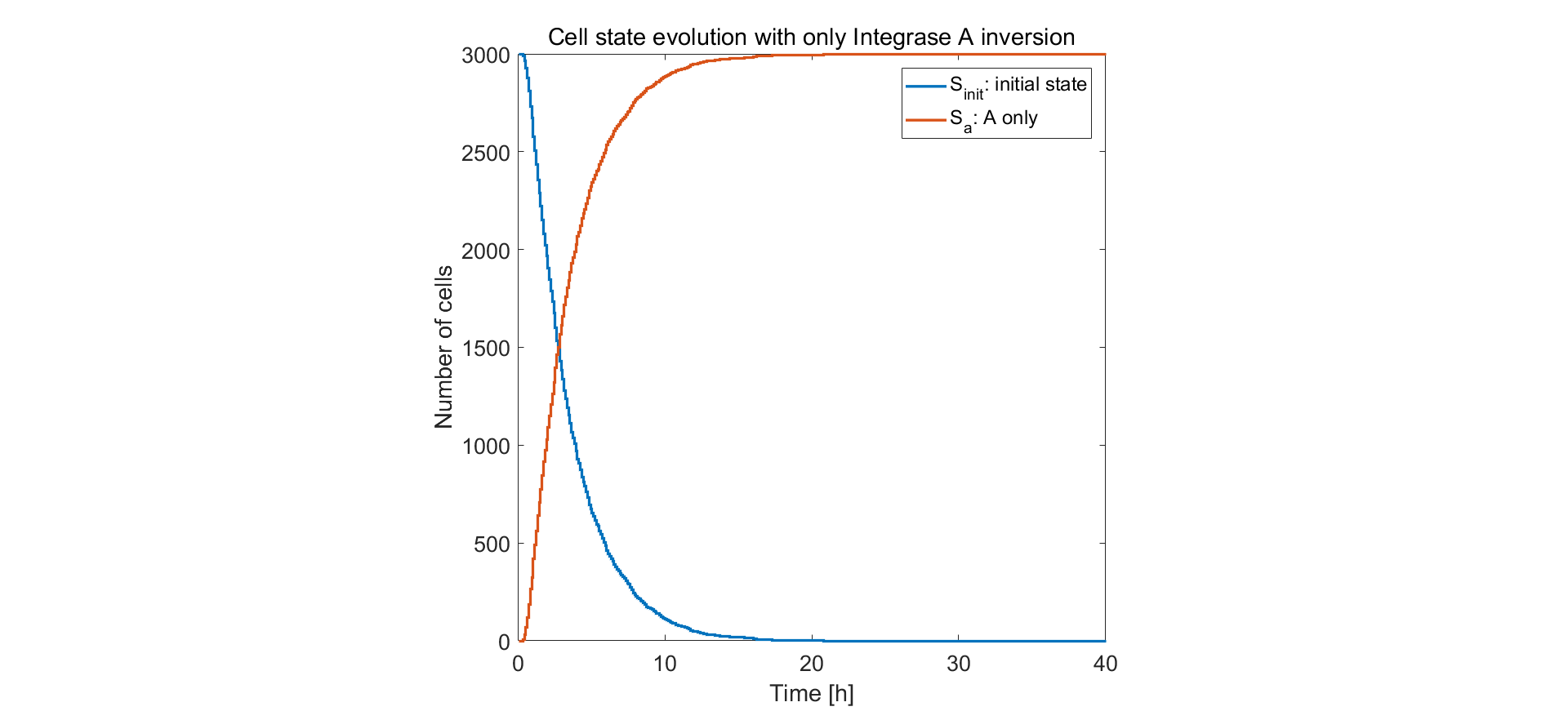Fig.1. Simulation result of only one pair of recombination sites on control unit circuit

The results for two pairs of recombination sites are shown below. We did two simulations. The first one (shown in the left figure), with two recombinases induced with no time separation, the system acts in an unsatisfactory way and approximately half of the cells are in the undesired state $$S_b$$. In the second one, inducing of intA and intB are separated with 5 hours. Then the system performs rather stably, with approximately 90% cells in desired state. This provides guidance for team members working in the lab.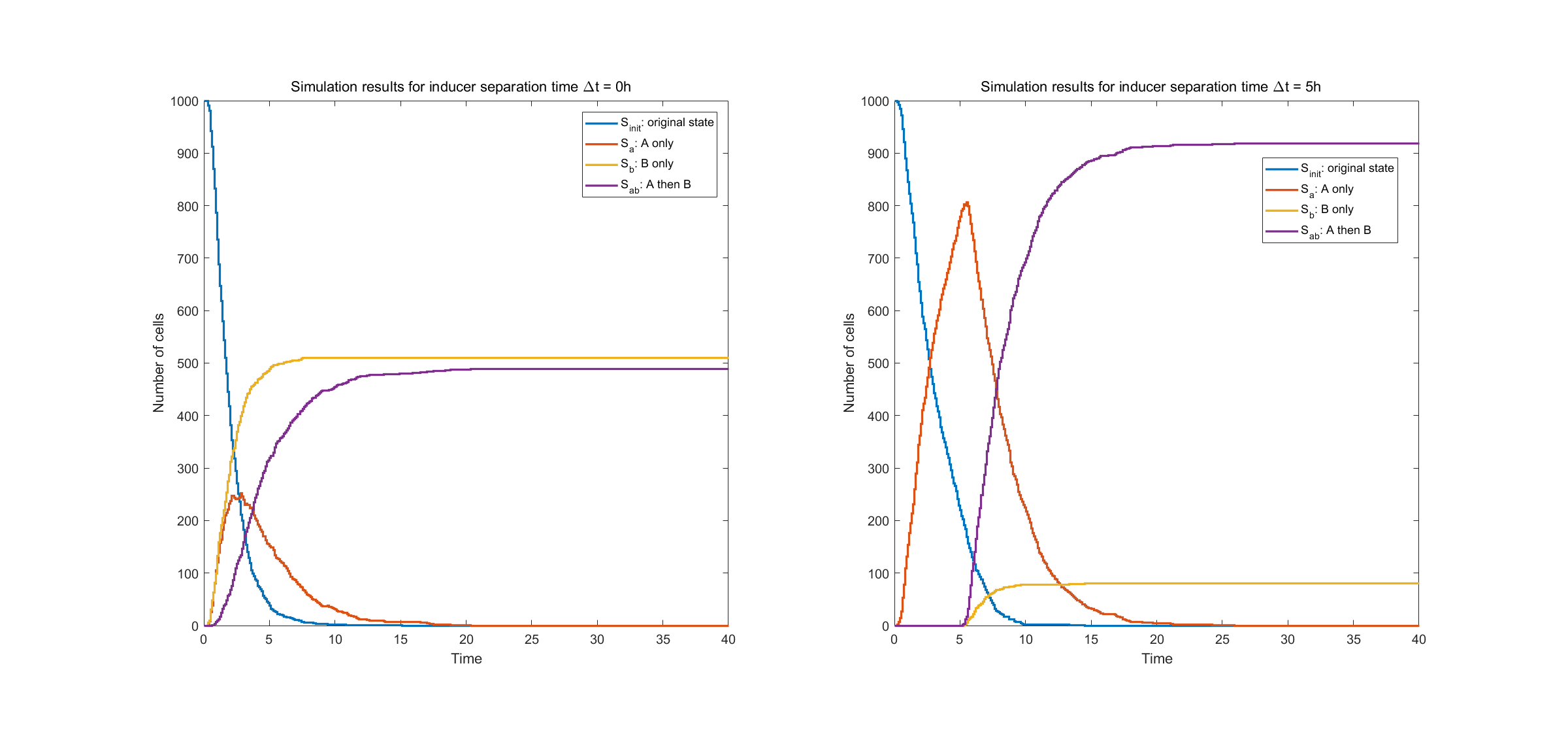Fig.2. Simulation result of two pairs of recombination sites

The left figure shows state evolution with no time separation in inducing events A and B. The right figure shows state evolution with time separation of $$\Delta t = 5h$$.

Finally we show specific cell transitions in Gillespie simulation. 100 cells are sampled from the population. Each vertical line denotes a cell state transition event (from $$S_{init}$$ to $$S_a$$, $$S_{init}$$ to $$S_b$$, from $$S_a$$ to $$S_{ab}$$). The last two subplots show the simulation results of protein expression in single cell.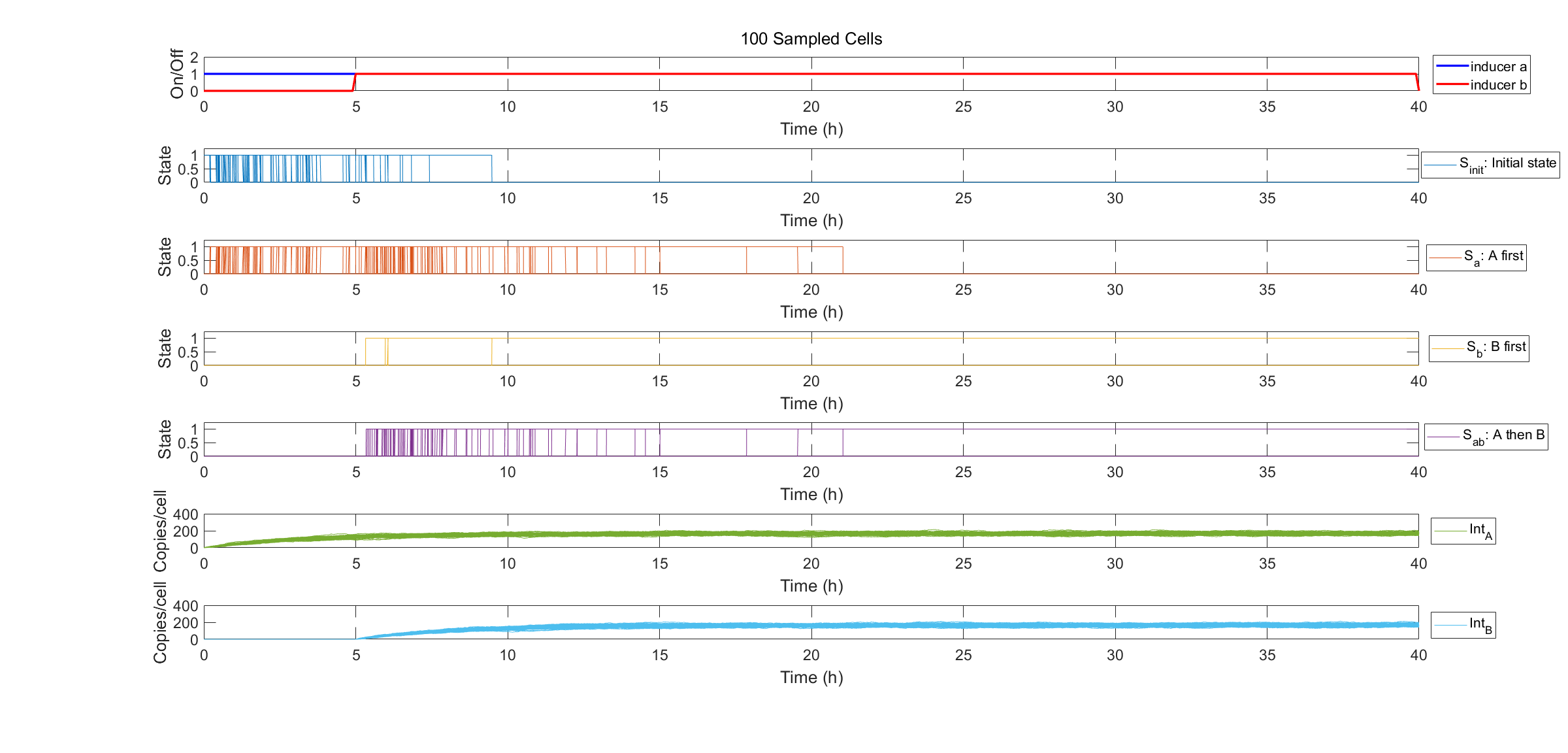Fig.3. Specification of state transitions in Gillespie simulation using 100 sampled cells.

## References

1. Elowitz, M. B., Levine, A. J., Siggia, E. D., & Swain, P. S. (2002). Stochastic gene expression in a single cell. Science, 297(5584), 1183-1186.
2. Ruess, J., Parise, F., Milias-Argeitis, A., Khammash, M., & Lygeros, J. (2015). Iterative experiment design guides the characterization of a light-inducible gene expression circuit. Proceedings of the National Academy of Sciences, 112(26), 8148-8153.
3. Gillespie, D. T. (1977). Exact stochastic simulation of coupled chemical reactions. J. phys. Chem, 81(25), 2340-2361.
4. Darzacq, X., Shav-Tal, Y., De Turris, V., Brody, Y., Shenoy, S. M., Phair, R. D., & Singer, R. H. (2007). In vivo dynamics of RNA polymerase II transcription. Nature structural & molecular biology, 14(9), 796-806.
5. Hsiao, V., Hori, Y., Rothemund, P. W., & Murray, R. M. (2016 ). A population‐based temporal logic gate for timing and recording chemical events. Molecular systems biology, 12(5), 869.
6. Chen, Y. J., Liu, P., Nielsen, A. A., Brophy, J. A., Clancy, K., Peterson, T., & Voigt, C. A. (2013). Characterization of 582 natural and synthetic terminators and quantification of their design constraints. Nature methods, 10(7), 659-664.
7. Rutherford, K., Yuan, P., Perry, K., Sharp, R., & Van Duyne, G. D. (2013). Attachment site recognition and regulation of directionality by the serine integrases. Nucleic acids research, 41(17), 8341-8356.
8. Singh, S., Ghosh, P., & Hatfull, G. F. (2013). Attachment site selection and identity in Bxb1 serine integrase-mediated site-specific recombination. PLoS genetics, 9(5), e1003490.

# Clock

## Intention of the Model

We attempts to develop a framework of biological sequential circuits that are programmable. The envisioned circuit is capable of both storing states in DNA and automatically running a series of instructions in a specific order. According to our design, our bio-flip-flop has two promoters, each triggered by an inducing signal. Therefore, if we would like to build an automatic triggering system for the bio-flip-flop, we need at least two signals in our clock. It is then self-evident to incorporate the classic structure in synthetic biology: the repressilator.

This model serves as a proof of concept for such implementation. We first constructed an ordinary differential equation (ODE) system to describe the repressilator, then we used two repressor proteins expressed by the repressilator to regulate the two promoters. Finally we adjusted parameters and gave a simulation result as a proof of the possibility of repressilator-driven bio-flip-flop.

## Biological Basis and Assumptions

The repressilator is a synthetic genetic regulatory network consisting of a ring-oscillator with three genes, each expressing a protein that represses the next gene in the loop. This network was designed from scratch to exhibit a stable oscillation which is reported via the expression of green fluorescent protein, and hence acts like an electrical oscillator system with fixed time periods. The repressilator consists of three genes connected in a feedback loop, such that each gene represses the next gene in the loop, and is repressed by the previous gene.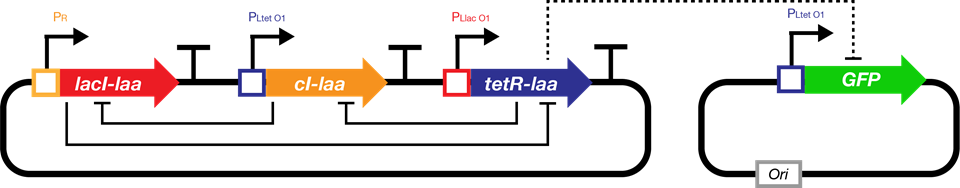Fig.1. The genetic structure of the repressilator network

We decided to implement our repressilator-triggered bio-flip-flop with two repressor proteins regulating the two promoters’ transcription. The basis of ODE system construction is illustrated as follows: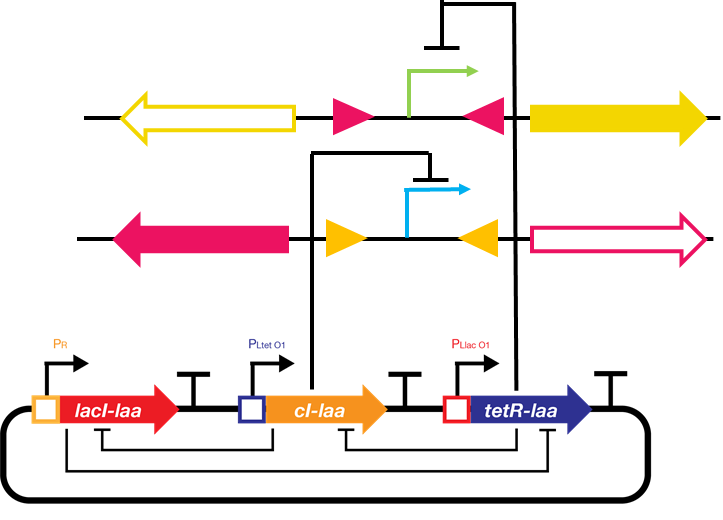Fig.2. Schematic drawing of the repressilator-driven bio-flip-flop

The upper promoter (X) is $$P_{LtetO}$$ and is repressed by tetR protein. The lower promoter (Y) is $$P_{R}$$ and is repressed by cI protein.Simulation of bio-flip-flop starts at a later time than repressilator because the expression levels of the repressor proteins at the beginning is not high enough.

## Equations and Parameters

### Repressilator

The following table lists the reactions in the repressilator network. X, Y, Z represent LacI, TetR and cI mRNAs, and PX, PY, PZ represent the corresponding proteins.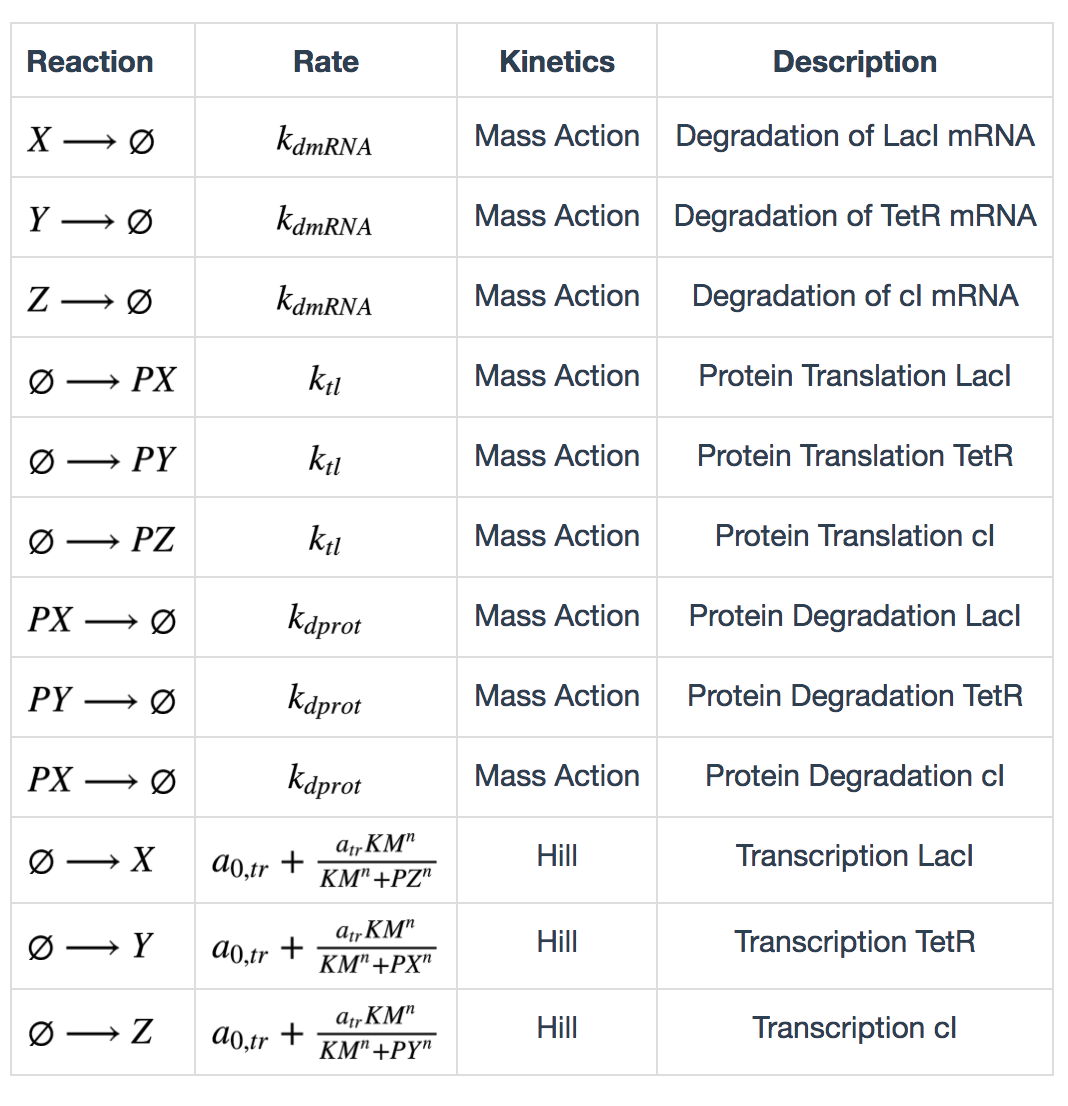Calculation of degradation rates are described as follows: $k_{dmRNA} = \frac{ln(2)}{\tau_{mRNA}}$ $k_{dprot} = \frac{ln(2)}{\tau_{protein}}$ $$\tau_{mRNA}$$ and $$\tau_{protein}$$ are half lives of mRNA and protein. Calculation of maximum transcription rate: $a_{tr} = 60(ps_a - ps_0)$ $$ps_a$$ is “tps active” and $$ps_0$$ is “tps_repressive”. Calculation of translation rate: $k_{tl} = \frac{eff}{t_{ave}}$ $$eff$$ is translation efficiency and $$t_{ave}$$ is average mRNA life time. $$t_{ave} = \frac{\tau_{mRNA}}{ln(2)}$$

### Transcription in bio-flip-flop

The transcription kinetics in the bio-flip-flop were modified to be repressed by repressors. These can be described by the following equations: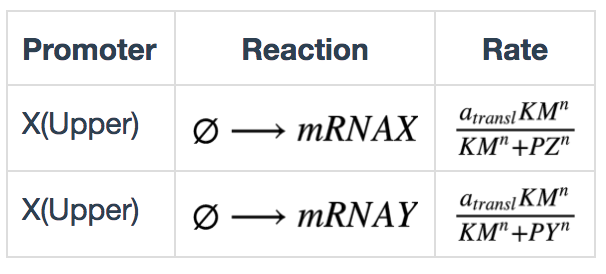## Simulation Results

We conducted our simulation using Tellurium, a Python-based simulation platform. We first adjusted the parameters protein half life and mRNA half life (equivalently transcription and translation of repressor proteins) to adjust oscillation period as the one observed in the experiment (See experiment).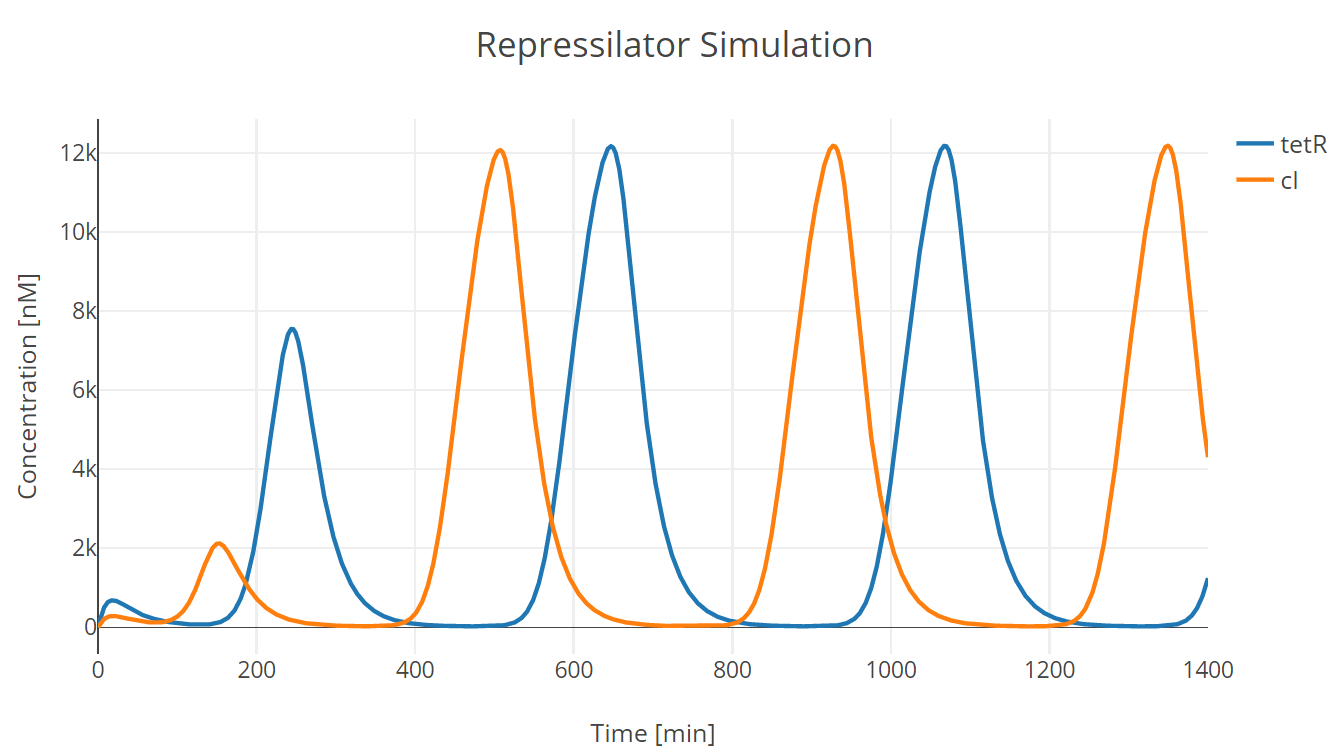Fig.1. Repressilator simulation results of tetR and cI protein

Then we incorporate the bio-flip-flop into the model, and we got the following simulation results: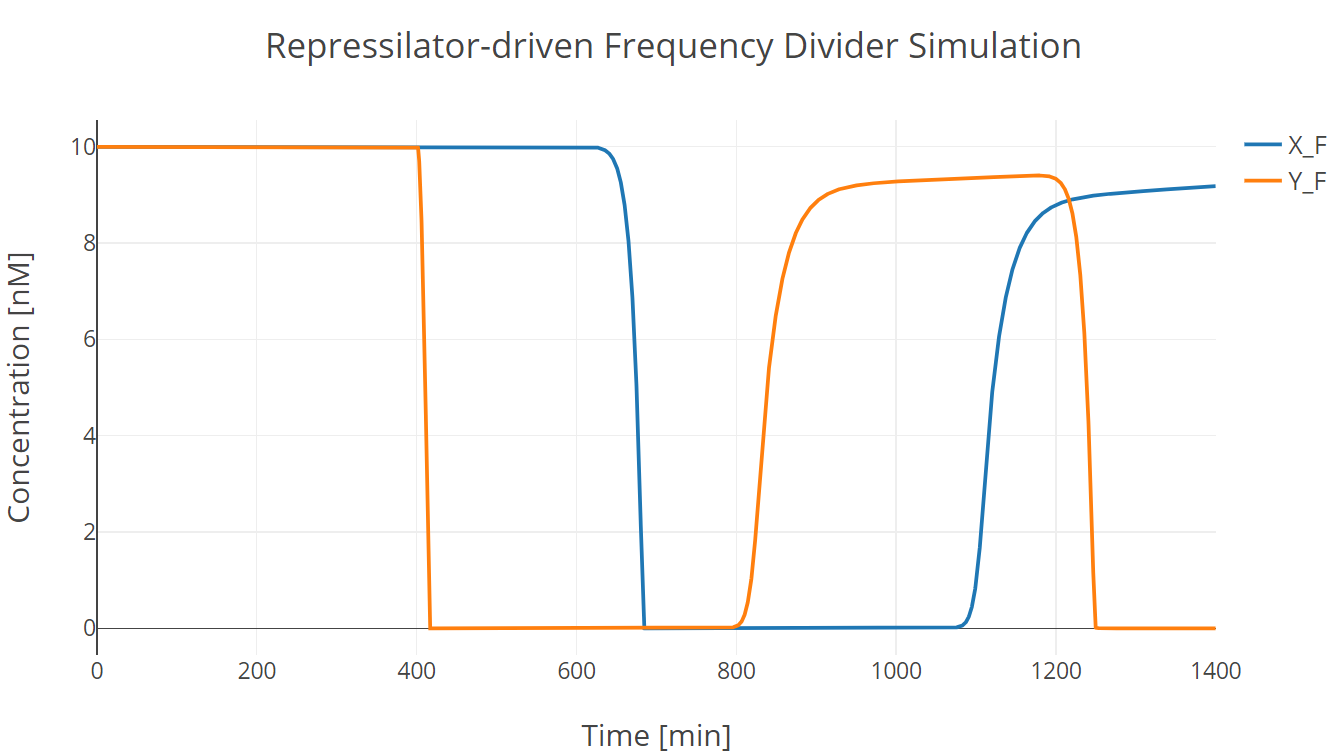Fig.2. Repressilator-driven frequency divider simulation with two trigger signal groups
X_F and Y_F have the same meaning as those described in Flip-flop section.

In this simulation experiment, the flip-flop lost approximately 10% of its initial state after two trigger signal groups.

## References

1. Elowitz, M. B., & Leibler, S. (2000). A synthetic oscillatory network of transcriptional regulators. Nature, 403(6767), 335-338.
2. https://wwwdev.ebi.ac.uk/biomodels/BIOMD0000000012
3. http://tellurium.analogmachine.org/

# Supplementary

The following are the parameters and tools we used in modelling and simulation. We share them in the interest of reproducibility.